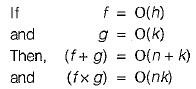Courses

# Algorithm Analysis And Asymptotic Notation (Basic Level) - 1

## 10 Questions MCQ Test Question Bank for GATE Computer Science Engineering | Algorithm Analysis And Asymptotic Notation (Basic Level) - 1

Description
This mock test of Algorithm Analysis And Asymptotic Notation (Basic Level) - 1 for Computer Science Engineering (CSE) helps you for every Computer Science Engineering (CSE) entrance exam. This contains 10 Multiple Choice Questions for Computer Science Engineering (CSE) Algorithm Analysis And Asymptotic Notation (Basic Level) - 1 (mcq) to study with solutions a complete question bank. The solved questions answers in this Algorithm Analysis And Asymptotic Notation (Basic Level) - 1 quiz give you a good mix of easy questions and tough questions. Computer Science Engineering (CSE) students definitely take this Algorithm Analysis And Asymptotic Notation (Basic Level) - 1 exercise for a better result in the exam. You can find other Algorithm Analysis And Asymptotic Notation (Basic Level) - 1 extra questions, long questions & short questions for Computer Science Engineering (CSE) on EduRev as well by searching above.
QUESTION: 1

### Sorting is useful for

Solution:

Sorting is useful for making search easier and efficient and in report generation but not minimize the space needed.

QUESTION: 2

### The order of an algorithm that finds whether a given Boolean function of ‘n’ variables, produces a 1 is

Solution:

In the worst case it has to check all the 2n possible input combinations, which is exponential.

QUESTION: 3

### The order of the binary search algorithm is

Solution:

Let there be ‘n' items to be searched, After the first search the list is divided into two, each of length n/2 . After the next search, 2 lists, each of length n/4  and so on. This successive division has to stop when the length of list becomes 1. Let it happen after k steps.
After the k steps,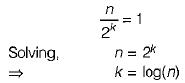Hence the order is log(n).

QUESTION: 4

For merging two sorted lists of sizes m and n into a sorted list of size m + n. Find out the time complexity of this merging process.

Solution:

Each comparison will append one item to the existing merge list. In the worst case one needs m + n -1 comparisons which is of order m+ n.

QUESTION: 5

A machine needs a minimum of 100 sec to sort 1000 names by quick sort. The minimum time needed to sort 100 names will be approximately

Solution:

In the best case quick sort algorithm makes O(nlog (n)) comparisons.
So 1000 x log (1000) = 9000 comparisons, which takes 100 sec.
To sort 100 names a minimum of 100 (log 100) = 600 comparisons are-needed.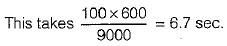QUESTION: 6

A machine took 200 sec to sort 200 names, using bubble sort. In 800 sec, it can approximately sort

Solution:

For sorting 200 names bubble sort makes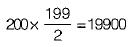comparisons. The time needed  for 1 comparison is 200 sec (approximately). In 800 sec it can make 80,000 comparisons. We have to find n, such that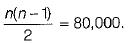Solving, n is approximately 400.

QUESTION: 7

Unrestricted use of goto is harmful, because it

Solution:

Unrestricted use of goto statement is harmful because it makes more difficult to verify programs i.e. use of goto can results in unstructured code and there can be blocks with multiple entry and exit which can cause difficulty which debugging of program.

QUESTION: 8

The recurrence relation that arises in relation with the complexity of binary search is

Solution:

Since, with every iteration of Binary Search, data set to be searched is divided into half of the original/earlier dataset. Also, same constant time is lapsed during each iteration. So, recurrence relation for Binary Search would be: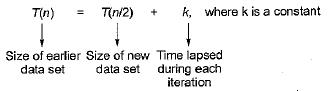QUESTION: 9

Consider the following two functions: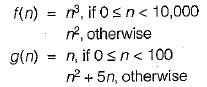Which of the following is/are true?
1. f(n) is O(n3)
2. g(n) is O (n3)
3. 0(f(n)) is same as O(g(n))
4. g(n) is O{n2)

Solution:

In this question total possibility is four but only two possibility will give solution and other two possibilities will not give solutions.
g(n) = O (f(n))
In case of 0 ≤ n < 100 & & 0 ≤n< 10,000
(it is also not possible)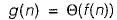in case of otherwise From the above two equations the conclusion is O(f (n)) is same as O(g(m)) and g(n) and f(n) will be O(n2).

QUESTION: 10

Suppose f, g, h, k : N→ N.
If f  =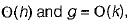then

Solution: